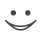Zu dieser Karteikarte gibt es einen kompletten Satz an Karteikarten. Kostenlos!

58
The zero curve is downward sloping. Define X as the 1-year par yield, Y as the 1-year zero rate and Z as the forward rate for the period between 1 and 1.5 year. Which of the following is true?
A.X is less than Y which is less than Z
B.Y is less than X which is less than Z
C.X is less than Z which is less than Y
D.Z is less than Y which is less than X